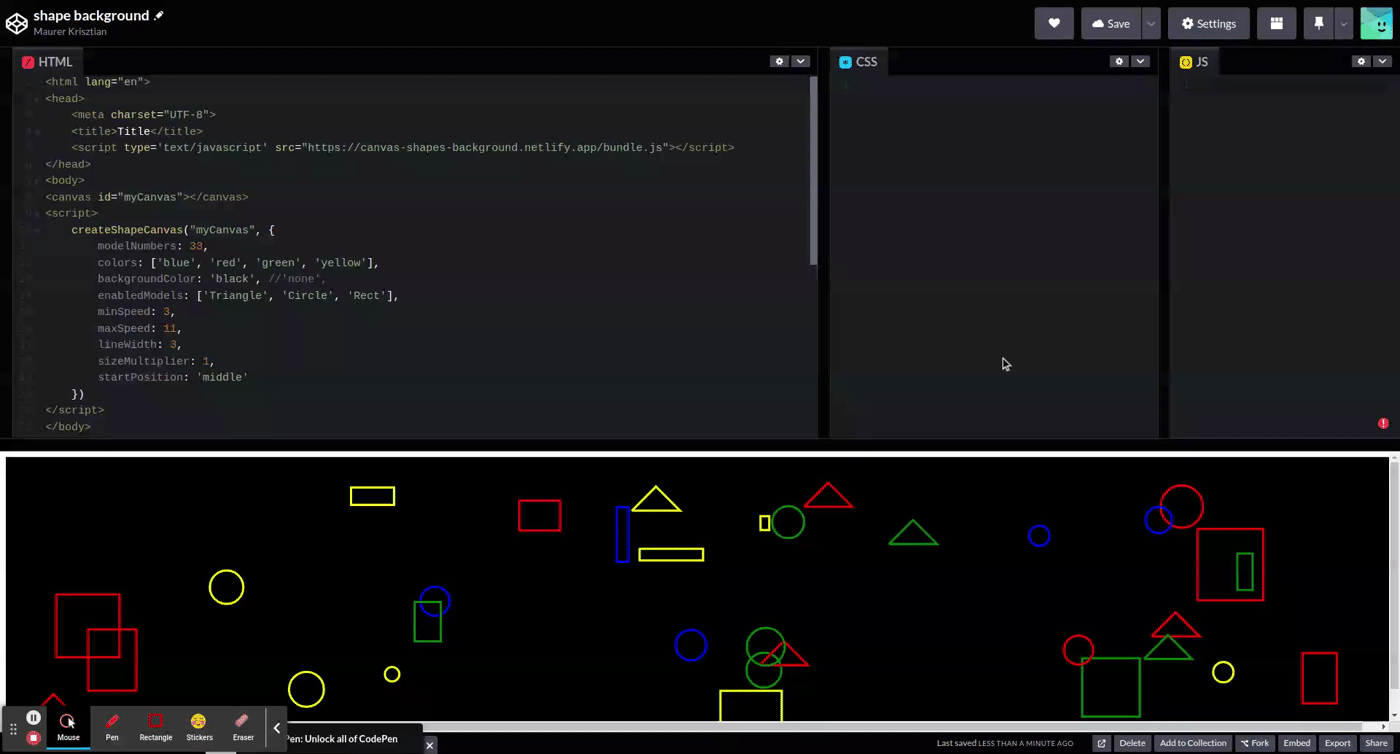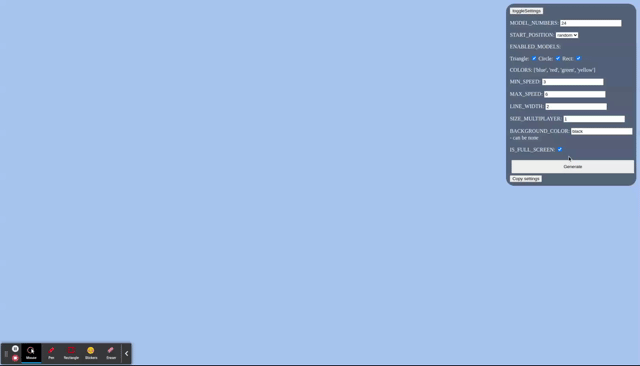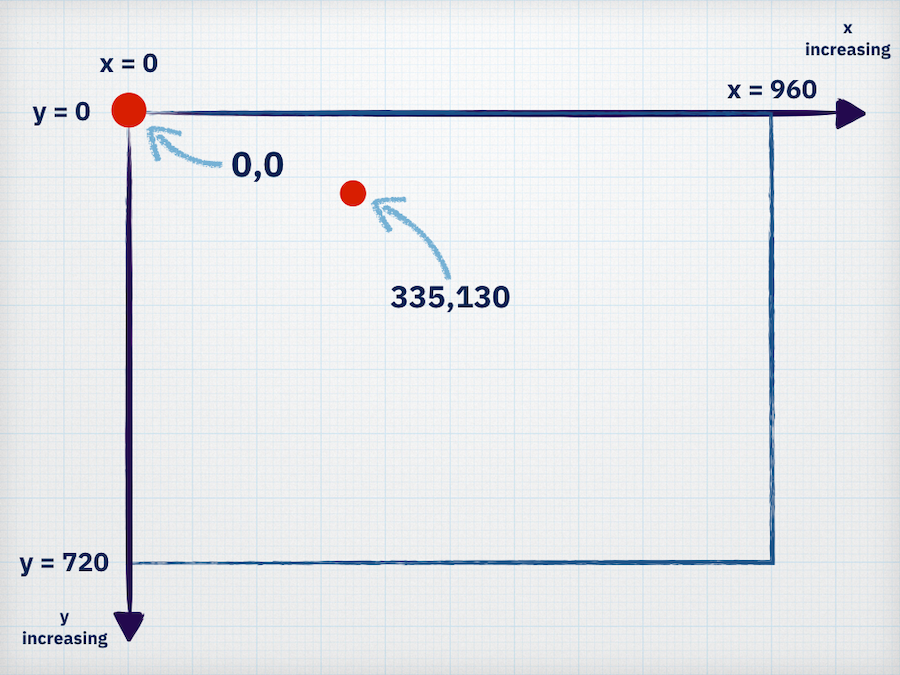# Canvas shapes background

HTML CanvasUIAnimation

### The goal of this project is to make a cool background with randomized shapes and to be customizable.

The goal of this project is to make a cool background with randomized shapes and to be customizable.

Simple example usage:https://codepen.io/maurerkrisztian/pen/rNdGerb

``````<html lang="en">
<meta charset="UTF-8">
<title>Title</title>
<script type='text/javascript' src="https://canvas-shapes-background.netlify.app/bundle.js"></script>
<body>
<canvas id="myCanvas"></canvas>
<script>
createShapeCanvas("myCanvas", {
modelNumbers: 11,
colors: ['blue', 'red', 'green', 'yellow'],
backgroundColor: 'black', //'none',
enabledModels: ['Triangle', 'Circle', 'Rect'],
minSpeed: 11,
maxSpeed: 11,
lineWidth: 3,
sizeMultiplier: 2,
startPosition: 'random' //  'middle' | 'random',
isFullScreen: true,
})
</script>
</body>
</html>``````

I added a configurator with the demo: https://canvas-shapes-background.netlify.app/

• Easily test different configs and copy the configured settings.“createShapeCanvas” has 2 arg

• canvas id
• config

# config

• startPosition: ‘middle’ | ‘random’ initial model position
• enabledModels: thies shapes will be generated randomly
• modelNumbers: generate this amount of models from “enabledModels” array
• colors: pisck random color from this array for shapes
• backgroundColor: canvas background color, can be “none”
• minSpeed: shape min speed
• maxSpeed: shape max speed
• lineWidth: shape border line width
• sizeMultiplier: multiply the generated size with this
• isFullScreen: fullscreen or element size

I used HTML Canvas.

I wanted to be easily extendable, so abstracted some thing:

#### Animator:

• it is responsible for setup every frame and call the modell update()

### Model:

• has 2 main responsibility:
• draw() - draw the shape,
• update()
• howto behave based on the current x,y position and
• xd, dy aka where to want to move and how fast. If you confused how dx, dy it works it basicly a vector. it has a direction (this will be the model direction) and a length (this will be the model speed).## Example model:

``````export class CircleModel implements IModel {
static MODEL_NAME = 'Circle'

color: string;

constructor(private x: number, private y: number, private dx: number, private dy: number, private radius: number, private lineWidth: number = Setup.CONFIG.lineWidth) {
this.color = Utils.pickRandomFromArray<string>(Setup.CONFIG.colors)
}

draw(context: CanvasRenderingContext2D) {
context.lineWidth = this.lineWidth;
context.beginPath();
context.arc(this.x, this.y, this.radius, 0, Math.PI * 2, false);
context.strokeStyle = this.color;
context.stroke();
context.lineWidth = DEFAULT_LINE_WIDTH;
}

update(context: CanvasRenderingContext2D) {
if (this.x > Context.canvasWidth - this.radius || this.x < 0 + this.radius) {
this.dx = -this.dx;
}
if (this.y > Context.canvasHeight - this.radius || this.y < 0 + this.radius) {
this.dy = -this.dy;
}

this.x = this.x + this.dx;
this.y = this.y + this.dy;

this.draw(context);
}
}``````

I wanted to baunce of from the side of the canvas so

Good to know where is the canvas side.

Coordinate system:So x(0) the left min position and x(canvasWidth) the right side posion, similarly with y.

``````        if (this.x > Context.canvasWidth - this.radius || this.x < 0 + this.radius) {
this.dx = -this.dx;
}``````

If the current position (center of the circle) greather than canvas width - radius than change the x direction

Similarly with y.

At the end we need to set the new position:

``````this.x = this.x + this.dx;
this.y = this.y + this.dy;``````

I made 3 model: Cirle, Rect, Triangle.

• create a model
``````    private static createRandomModel() {
const speed = Utils.generateRandom(Setup.CONFIG.minSpeed, Setup.CONFIG.maxSpeed)
const randoModelName = Utils.pickRandomFromArray(Setup.CONFIG.enabledModels)
switch (randoModelName) {
case CircleModel.MODEL_NAME:
return this.createRandomCircle(speed)
break;
case TriangleModel.MODEL_NAME:
return this.createRandomTriangle(speed)
break;
case RectModel.MODEL_NAME:
return this.createRandomRect(speed)
break;
default:
}
}``````

## Howto create importable script?

I just added the init function to the window so you can import the bundle script and call this function.

``window.createShapeCanvas = createShapeCanvas``